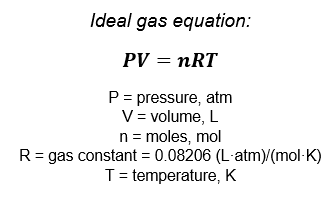# Problem: Magnesium can be used as a "getter" in evacuated enclosures to react with the last traces of oxygen. (The magnesium is usually heated by passing an electric current through a wire or ribbon of the metal.) If an enclosure of 0.462 L has a partial pressure of O2 of 3.6 × 10−6 torr at 28oC, what mass of magnesium will react according to the following equation: 2 Mg(s) + O2(g) → 2 MgO(s)?

###### FREE Expert Solution

First, calculate how many moles of O2(g) is present in the enclosure using the ideal gas equation. Then do a mole to mole comparison to determine the amount of Mg(s) that will react with O2(g):Given:

97% (34 ratings)###### Problem Details

Magnesium can be used as a "getter" in evacuated enclosures to react with the last traces of oxygen. (The magnesium is usually heated by passing an electric current through a wire or ribbon of the metal.) If an enclosure of 0.462 L has a partial pressure of O2 of 3.6 × 10−6 torr at 28oC, what mass of magnesium will react according to the following equation: 2 Mg(s) + O2(g) → 2 MgO(s)?

Frequently Asked Questions

What scientific concept do you need to know in order to solve this problem?

Our tutors have indicated that to solve this problem you will need to apply the Gas Stoichiometry concept. You can view video lessons to learn Gas Stoichiometry. Or if you need more Gas Stoichiometry practice, you can also practice Gas Stoichiometry practice problems.